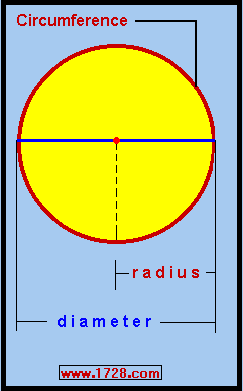A Quick History of PI   π
 If you want to calculate the curved distance around a circle (the circumference), it would not be easy to measure with a ruler.It is much easier to measure the distance of the line (diameter) passing through the center of a circle which also divides the circle into 2 equal sections. The only problem here is determining a number (called π which when multiplied by the diameter would yield the circumference. The early values of "pi", obtained by physically measuring circle circumferences then dividing this by their diameters were not very accurate and no doubt were not much better than 3. Calculating "pi" by mathematical means is exact but extremely difficult. The first person to calculate "pi" mathematically was Archimedes (287 — 212 BC) who did this by calculating the perimeter of a 96 sided polygon. He concluded that the value of "pi" was between (223 / 71) (or 3.1408) and (22 / 7) (or 3.1429). If we take the average of those 2 numbers we get 3.14185 which is extremely close to the actual value of 3.14159. As can be seen, Archimedes' calculation was accurate to 3 decimal places (4 significant figures). As the centuries passed, mathematicians did not get much more accurate values of "pi". Also, for the next several centuries, mathematicians wondered if the number "pi" could be a repeating decimal, such as 1/7 = .142857142857142857... Finally, the Dutch mathematician Ludolph Van Ceulen (1540 - 1610) in 1596 calculated "pi" to 20 decimal places and then in 1615 in a paper published posthumously showed his calculation to 35 decimal places. (This required his calculating the perimeter of a polygon with 262 (≈ 4.6 quintillion) sides!!!) 3.14159265358979323846264338327950288 Now, for the first time, the world had an extremely accurate version of "pi". If nothing else, Ludolph's 36 significant figure value of "pi" showed that the chances of "pi" being a repeating decimal were extremely remote. As for calculating "pi", instead of using geometric methods involving polygons, more accurate values were obtained by using infinite series and calculus. In the next several centuries, more accurate values were produced until 1873, when British mathematician William Shanks calculated "pi" to 707 places. This held the record until 1946 when it was discovered that Shanks made a mistake in calculating the 528th decimal place. Up until the 1940's, all "pi" calculations had been done with paper and pencil. Now, with the advent of computers, the number of decimal places has increased phenomenally. YEAR   DECIMAL   PLACES 1949   >2,000 1958   >10,000 1961   >100,000 1973   >1,000,000 1989   >1,000,000,000 2002   >1,000,000,000,000 2011   >10,000,000,000,000 2016   >22,000,000,000,000 2019   31,415,926,535,897   (note that this number is "pi" * 1013). This value of "pi" was announced on March 14, 2019, ("Pi Day"). This was calculated by Emma Haruka Iwao, an employee of Google, Japan. 2020   50,000,000,000,000   50 trillion digits! Announced on January 29, 2020 and calculated by Timothy Mullican of Alabama, USA. 2021   62,800,000,000,000   62.8 trillion digits! Calculated by The University of Applied Sciences in Graubünden, Switzerland. New pi calculation record Formulas for Calculating Pi There have been many formulas developed for calculating pi. For example, this formula which just uses simple arithmetic, at first, looks rather easy to solve: π = 4   - 4/3   + 4/5   - 4/7   + 4/9   - 4/11 ... However, carrying this out to -4 / 99 yields a "pi" vaue of 3.12159465259101... Considering that you had to add 50 fractions to get a value of pi accurate to just one decimal digit, we can see that this formula approaches the actual value of pi very slowly. The formula below converges on "pi" much more quickly: π = 16 • arc tan (.2)   -4 • arc tan (⅟ 239) The problem with the above formula is that we have to calculate 2 transcendental numbers (the arc tangent values) which is not an easy task to do. To read more about calculating the value of "pi" click the link here. Just how accurate a value of "pi" do any of us require? According to Marc Rayman, NASA only requires a "mere" 15 decimal places of "pi" for their most accurate work. It is astounding to think that in 1596, Ludolph calculated a 20-decimal value of "pi" that is 100,000 times more accurate than even NASA requires! And just to ensure that the importance of π is not forgotten, March 14th has been unofficially designated a national holiday. It is also Albert Einstein's birthday - March 14, 1879. Sadly, the legendary physicist, Stephen Hawking, passed away on March 14, 2018.